# Algorithm, computational complexity of an

Jump to: navigation, search

A function giving a numerical estimate of the difficulty (amount of time/storage involved) of the process of application of an algorithm to the inputs. A more precise definition of the computational complexity of an algorithm is the concept of a cost function (step-counting function) — defined as a decidable relation between the objects to which the algorithm is applicable and the natural numbers, and which has a range of definition coinciding with the range of applicability of the algorithm.

Usually, the time and space characteristics of algorithmic processes are considered. Thus, for a Turing machinethe time cost function (duration of work)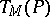is the numberof cycles of work ofrequired for the conversion of the initial configuration of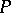into the final configuration; the memory cost function (or space function)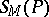is defined as the number of cells on the tape scanned by the head of the machine. Time and storage costs for normal algorithms (cf. Normal algorithm), iterative arrays, multi-head and multi-tape Turing machines, etc., are defined in a similar manner.

The common feature of such cost functions is the fact that there exists an effective procedure establishing for any algorithm(i.e. in particular for a Turing machine or, rather, for its program), any inputand any non-negative integer, whether or not the process of applyingtowill terminate with complexity. This observation leads to the abstract theory of computational complexity . An effective procedureis called a computational measure if: 1) it always ends in the result 0 or 1 when applied to triplets of the form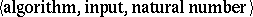; and 2) it has the property that for any algorithmand any inputthe equality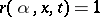is true for not more than one natural number, thisexisting if and only if the process of application oftoeventually terminates. The cost function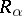with respect to the measureforis introduced if and only if, and then.

This last equality is equivalent to the statement "the computational complexity a on x is t (in the measure r)" .

Given some computational measure, one can consider the complexity of computation of a given function— for example, that of finding an algorithmwhich computes"better than other algorithms" . However, as exemplified by the speed-up theorem (see below), such a formulation is not always well-posed. The real problem may be the description of the rate of growth of the cost function, wherecomputes. For example, find upper and lower bounds for the computational complexity of, i.e. two functions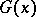and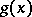such that there exists a computationfor the functionfor which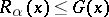, and such that for any algorithmwhich computesthe function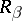in a certain sense majorizes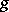.

Another important branch of the theory of computational complexity is the study of complexity classes — sets of functions for which there exist computations with a complexity not exceeding some bound out of the set of bounds which defines the class. This branch also includes problems of comparative computational complexity for various types of algorithms (automata): the transition to another algorithmic system and another measure of complexity is usually equivalent to considering a new measure for the first algorithmic system.

Below a few fundamental results are given which are independent of the choice of measure of complexity (and of the formalization), as long as there exists, for example, an effective mutual simulation of the algorithms of the type under consideration and ordinary Turing machines. (For the sake of simplicity, it is assumed that the subject is the computation time of Turing machines computing functions from non-negative integers to non-negative integers.) Letandbe computable integer-valued functions (cf. Computable function) on the domain of the algorithms, and letbe a function defined on this domain which can assume two values only — say, 0 and 1.

First, there exist functions whose computation may be as complex as one pleases. More exactly, for any functionthere exists a computable functionsuch that for any algorithmwhich computes, the inequality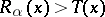is false only in a finite number of cases.

Secondly, there exist functions, any computation of which may in principle be improved as much as one pleases. More precisely, the speed-up theorem is valid: For any function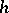(e.g.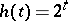) it is possible to find a computable functionsuch that for any algorithmwhich computesit will always be possible to find (no effective procedure is assumed) an algorithmwhich also computesand such that for all(except for a finite set)In casethis yieldsfor almost all.

For the computational measures of time and space (for Turing machines), the result of the speed-up theorem is valid for most computations in the following form: Ifis computable with complexity, it is also computable with complexity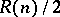(one says thatis computable with complexity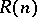if, for somewhich computes it,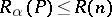for all wordsof lengthin the alphabet under consideration). For this reason time and space computational complexities of concrete functions are often order of magnitude estimates. Some specific results are quoted below.

The time to decide the equality of words (of length) is of orderfor Turing machines and normal algorithms; any property of words which may be decided on Turing machines in a time asymptotically less than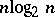can also be decided in time; computations by a Turing machine of time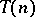(with the order of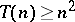) can be simulated by a Turing machine with space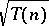. Furthermore, the multiplication of two-digit numbers on multi-tape Turing machines can be performed in time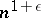, but not in time order, but iterative arrays can achieve this in real time, i.e. the-th digit of the product is produced before the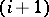-th digits of the factors are inputted. When algorithmic processes of one type are modeled by other processes, the computational complexity usually changes. Thus, a reduction in the dimension of the iterative arrays results in longer computation time. Multi-head Turing machines and multi-tape Turing machines can simulate each other in real time. Many types of algorithms can be simulated on Turing machines, such that the computation time increases polynomially (usually quadratically) with an insignificant increase in space complexity (usually, an additional constant factor).

The complexity approach is useful in studying certain hierarchies of classes of general recursive functions (cf. General recursive function), in connection with their logical and algebraic features. For instance, primitive recursive functions coincide with the functions computable by Turing machines in space bounded by a primitive recursive function. The existence of arbitrarily complex functions is established by diagonalization methods. Natural analogues for such lower bounds on computational complexity were found for the validity problem in certain decidable theories and in areas related to mathematical linguistics. However, for many problems of practical importance the problem of obtaining non-trivial lower bounds presents considerable difficulties. This is the case, in particular, for certain combinatorial problems which are formalized (in one variant) as problems of finding, out of all words of a given word length, those which satisfy a predicate computable in polynomial time. One can also mention the complexity of the computations performed by non-deterministic automata and probabilistic automata (cf. Automaton, probabilistic). In the non-deterministic case the computational device can choose both ways when presented with a choice of how to continue the computation, by "splitting" into two independent copies, each of which has the same capabilities as its parent. Its computational complexity is understood to mean the complexity of the "simplest" successful computation trace. In the probabilistic case, the actual course of the computation is determined not only by the program and the argument, but also by a random sequence of numbers, and the result should coincide, to a great degree of probability, with the value of the function being computed. In both cases the computational complexity can sometimes be proved to be less than that of deterministic computations for the same problem.

The quality of algorithms is evaluated not only by their computational complexity, but also by the complexity of description an algorithm (cf. Algorithm, complexity of description of an). In some publications, both these approaches are combined.